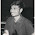## Multi-Choice Questions [MCQ] with Answers from Batteries

 A battery consists of
(a) A cell
(b) A circuit
(c) A generator
(d) A number of cells

 The terminal potential difference of a cell of e.m.f. 2V and internal resistance 0.1Ω when supplying a current of 5A will be
(a) 1.5V
(b) 2V
(c) 1.9 V
(d) 2.5V

 Five cells, each with an e.m.f. of 2V and internal resistance of 0.5Ω are connected in series. The resulting battery will have
(a) An e.m.f. of 2V and an internal resistance of 0.5 Ω
(b) An e.m.f. of 10V and an internal resistance of 2.5 Ω
(c) An e.m.f. of 2V and an internal resistance of 0.1 Ω
(d) An e.m.f. of 10V and an internal resistance of 0.1 Ω

 If the five cells of question 3 are connected in parallel the resulting battery will have
(a) An e.m.f. of 10V and an internal resistance of 2.5 Ω
(b) An e.m.f. of 2V and an internal resistance of 0.5 Ω
(c) An e.m.f. of 2V and an internal resistance of 0.1 Ω
(d) An e.m.f. of 10V and an internal resistance of 0.1 Ω

 Which of the following statements is false?
(a) A Leclanché cell is suitable for use in torches
(b) A nickel–cadmium cell is an example of a primary cell
(c) A secondary cell may be recharged after use
(d) When a cell is being charged its terminal p.d. exceeds the cell e.m.f.

 Which of the following statements is false?
When two metal electrodes are used in a simple cell, the one that is higher in the electrochemical series:
(a) Tends to dissolve in the electrolyte
(b) Is always the negative electrode
(c) Reacts most readily with oxygen
(d) Acts an an anode

 Five 2V cells, each having an internal resistance of 0.2Ω are connected in series to a load of resistance 14 Ω. The current flowing in the circuit is
(a) 10A
(b) 1.4A
(c) 1.5A
(d) 2/3A

 For the circuit of question 7, the p.d. at the battery terminals is
(a) 10V
(b) 9(1/3)V
(c) 0V
(d) 10(2/3)V

 Which of the following statements is true?
(a) The capacity of a cell is measured in volts
(b) A primary cell converts electrical energy into chemical energy
(c) Galvanising iron helps to prevent corrosion
(d) Apositive electrode is termed the cathode

 The greater the internal resistance of a cell
(a) The greater the terminal potential difference.
(b) The less the e.m.f.
(c) The greater the e.m.f.
(d) The less the terminal potential difference.

 The negative pole of a dry cell is made of
(a) Carbon
(b) Copper
(c) Zinc
(d) Mercury

 The energy of a secondary cell is usually renewed
(a) By passing a current through it
(b) It cannot be renewed at all
(c) By renewing its chemicals
(d) By heating it

 Which of the following statements is true?
(a) A zinc carbon battery is rechargeable and is not classified as hazardous
(b) A nickel cadmium battery is not rechargeable and is classified as hazardous
(c) A lithium battery is used in watches and is not rechargeable
(d) An alkaline manganese battery is used in torches and is classified as hazardous
5:49 PM

1.2.